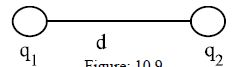Physics

# Explain Coulomb’s Law

Law: The forces of attraction or repulsion between two charged bodies in particular medium is directly proportional to the product of the charges and inversely proportional to the square of the distance between them and the force acts along the straight line connecting them. Suppose, two charges q1 and q2 are at a distance d from each other [Fig]. The force of attraction or repulsion between the two charges depends on,

1. quantity of charges.
2. distance between two charges.
3. the nature of the medium between the two charges.

Scientist Coulomb states a law about the force of attraction or repulsion between the two charges. This is called Coulomb’s law.

If the force of attraction or repulsion between these two is F, then according to Coulomb’s law,F ∞ q1q/d2

Or, F ∞ Cq1q/d2

Here C is a constant of proportionality. Its value in vacuum is 9 x 109 Nm2 C-2. Sometimes it is called coulomb’s constant.

Unit of charge: The unit of charge is coulomb (C). It is a derived unit. Coulomb is defined from ampere. If 1 ampere (1 A) current flows through a conductor for 1 second (1 s), then the amount of charge that passes through any cross section of the conductor is called coulomb (1 C).